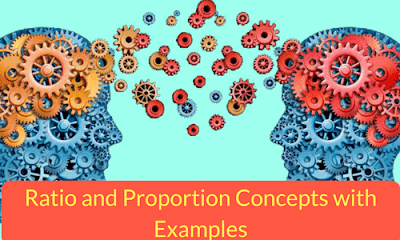# Ratio and Proportion Concepts with Examples## RATIO:

• Ratio is the relation in which one quantity bears to another of the same kind, the comparison being made by considering what multiple, part or parts; one quantity is of the other.
• The ratio of two quantities ‘a’ & ‘b’ is represented as a: b & read as ‘a is to b’.
• In a ratio a: b, ‘a’ is called antecedent & ‘b’ the consequent. Since the ratio expresses the number of times one quantity contains the other, it’s an abstract quantity & thus has no units.
• A ratio a: b can also be expressed as a/b. So if two terms are in the ratio 2:3, we can say that their ratio is 2/3. If two terms are in the ratio 2, it means that they are in the ratio of 2/1 i.e., 2:1
“A ratio is said to be a ratio of greater inequality or less inequality or of equality according as the antecedent is greater than, less than or equal to the consequent”.

We observe that a ratio of a greater inequality is diminished & a ratio of lesser inequality is increased by adding the same positive quantity to both the terms.
In a: b, (where a, b are positive)

If a<b, then (a+x): (b+x) > a: b and
If a>b, then (a+x): (b+x) < a: b

In case of 2:3, we observe that (2+1): (3+1) i.e., 3:4 (i.e., 0.75) is greater than 2:3(i.e., 0.66)
If a/b=c/d=e/f, then each of these ratios is equal to (a+c+e)/ (b+d+f).

## Examples

### Example 1

Three numbers are in the ratio of 4:7:11. The sum of the three numbers is equal to 88.What is the difference b/w the largest & smallest of the three?
Solution:-
⇒Let the three numbers be 4x, 7x & 11x.
⇒ 4x+7x+11x=22x=88
⇒X=4
Therefore, the difference b/w the largest & the smallest of the three= (11-4)4 =28

### Example 2

Four persons P, Q, R & S divided Rs 6255/- among themselves in the ratio of 7:9:11:18. How much amount did Q & R together get?
Solution:Let the amounts with P, Q, R & S be 7a, 9a, 11a & 18a respectively.
⇒7a+9a+11a+18a=45a=625545a=6255⇒ a= 6255/45=139
Q & R together got,
⇒ 9(139) +11(139) =2780

## PROPORTION

When two ratios are equal, the four quantities involved in the two ratios are said to be proportional i.e., if a/b=c/d, then a, b, c & d are proportional. This is represented as a: b:: c:d & is read as ‘a is to b (is) as c is to d’.
Definition: A proportion is an equation stating that two ratios are equal.
For example a:b = c:d

When a, b, c & d are in proportion, then the numbers a & d are called the EXTREMES & the numbers b & c are called the MEANS. As a:b::c:d implies a/b=c/d by cross multiplication, we have ad=bc, hence we write it as

‘Product of the MEANS=Product of the EXTREMES’### Properties of ProportionWhenever we know a/b=c/d, then we can write
(a+b)/ (a-b) = (c+d)/(c-d).

The converse of this is also true-whenever we know that (a+b)/ (a-b) =(c+d)/(c-d), then we can conclude that a/b=c/d.
If three quantities a, b &c are such that a: b:: b: c, then we say that they are in CONTINUED PROPORTION. We also get b²=ac.

### Example 1

A and B together have Rs. 2000. If 4/5 of A's amount is equal to 2/5 of B's amount, how much amount does B have?
Solution:-
⇒ 4/5 A= 2/5 B
⇒ A= [(2/5) X (5/4)]B
⇒ A=(1/2)B
⇒  A/B=1/2
⇒ A:B= 1:2
Therefore, B’s share = [2000x2/3] =Rs 1333.33/-

### Example 2

In a mixture 90 litres, the ratio of milk and water 2: 1. If this ratio is to be 1: 2, then the quantity of water to be further added is?
Solution:-
⇒ Quantity of milk =  [90 x  (2/3)] =60 litres.
⇒ Quantity of water in it= [90-60]=30 litres
⇒ New ratio = 1:2
Let quantity of water to be added further be x litres
⇒ Then,milk : water = 60/(30+x)
⇒ 60/(30+x) = 1/2
⇒ 30+x=120
⇒ X=90 litres

## Practice problems

1) If 0.75: x:: 5: 8, then x is equal to?

2) The fourth proportional to 5, 8, and 15 is?

3) Three numbers are in the ratio of 11:13:15. The largest number is 36 less than the sum of other two numbers. What is the smallest of the three numbers?

4) A sum of Rs 5640 is divided among P, Q & R in the ratio 13:16:18. How much amount did R get more than P?

1) 1.20
2) 24
1) 66
2) 600